Need Help? Chat with us

# Aggregate Function in Excel

## Aggregate function in Excel

The Aggregate function in Excel allows you to apply function like SUM, AVERAGE, COUNT, MIN, MAX, etc. Excel functions like Sum, Average, Count, Min, Max cannot function if the range includes error. However, if you use Aggregate function along with these function, your problem will be fixed.

The Aggregate function is an in-built function in Excel. It is categorized under the Math/Trig Function. It can be used as an aggregate function in Excel. And can be entered as a part of the formula.

## Special Offer for First Time Users

If you are the first time user and want to learn Microsoft office skills, then you can avail this special offer discount on your email.At glance, Aggregate function will look like SUBTOTAL function. This function is much more powerful. The Aggregate function has two Syntax forms:

1. Reference Form

2. Array Form

### 1. Reference Form

The syntax of Aggregate function with Reference Form is

AGGREGATE(function_num, options, ref1, …)

where,

Function_num: You can specify any number between 1 to 13. Each number represents a function. The list of this of the function number along with the name as explained in the image below. This argument is compulsory.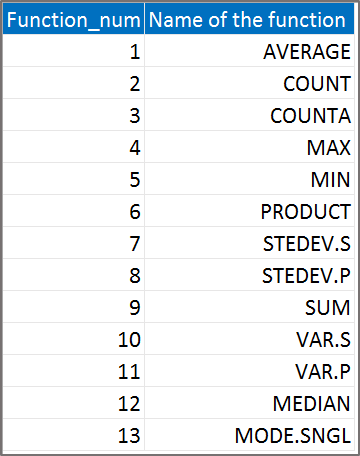Options: This argument specifies which value to be ignored in the evaluation. There is a specific number assigned to each values that are to be ignored. The image below explains you the options available.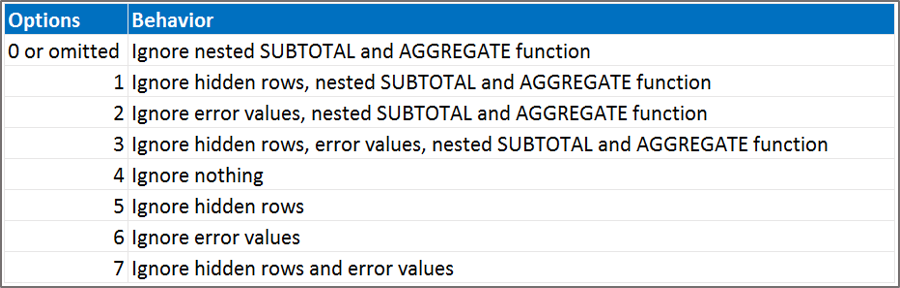Ref1: This argument is also compulsorily required while entering the formula. This arguments specifies for which range you want to use the Aggregate function.

### 2. Array Form

The syntax of Aggregate function with Array Form is:

AGGREGATE(function_num, options, array, k)

Function_num: You can specify any number between 14 to 19. Each number represents a function. The list of this function number along with the name is explained in the image below. This argument is compulsory.Options: This argument specifies which value is to be ignored in the evaluation. There is a specific number assigned to each values that are to be ignored. The image below explains you with the various options available.Array: This argument too is compulsory. It works like a reference to a range of cells for which you want to use the aggregate function.

K: Each function in this form is required with a second argument. This argument is compulsory to enter while using array function.

Let’s take a look on how you can use the aggregate function in Excel. Below is the example and how aggregate function is used along with the other functions.

In our example, let’s take into consideration the Sum function to explain the Aggregate function in Excel using The Reference formula.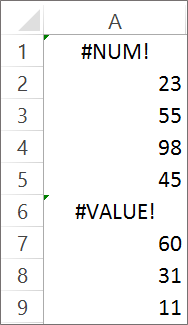We want to calculate the Aggregate function for the above example using the reference formula.

Here the arguments will be:

Function_Num: We will enter number 9 which represents Sum.

Options: We will Ignore the Error value so we will enter number 6.

Ref1: This will be the range for which you want to calculate aggregate functions. Here it is A1:A9.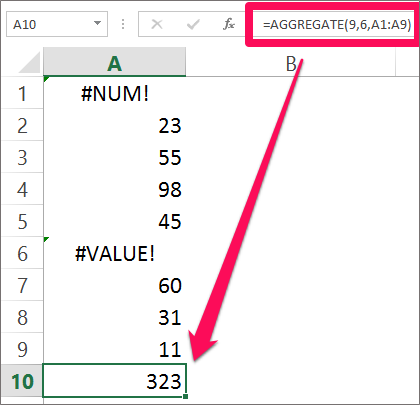The result will be 323.

Let us take one more example that will explain you how to calculate Aggregate function using the Array form.

We will use the aggregate functions to calculate the small second smallest number in the example.The arguments will be entered as

Function_name: 15 which is a representative of Small.

Options: Ignore Error Value which will be 6

Array: The range for which we want to calculate aggregate formula. Here the range is A1:A9

K: This is the second argument. Here we want to calculate the smallest number or we will enter 2.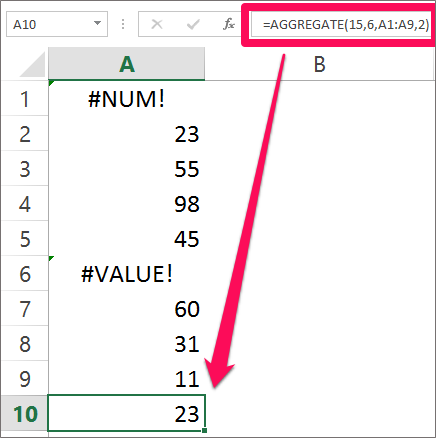The result will be 23.

You can use the other function as well to calculate the Aggregate functions in Excel. You will now agree to the fact that those Aggregate function is like Subtotal function in Excel. But it is more powerful.

Note: Aggregate is designed to work with the data in columns, so when you give reference as horizontal range Aggregate will not ignore values in hidden columns. It only ignores hidden rows.

Hope you like our tutorial on this for more cool and amazing trick of excel Like our Excel Superstar Facebook Page and subscribe to our Excel Superstar YouTube channel. Excel Superstar is the leading online training company, which provides Online Excel Course in Hindi. Connect with us and become an Excel Superstar.